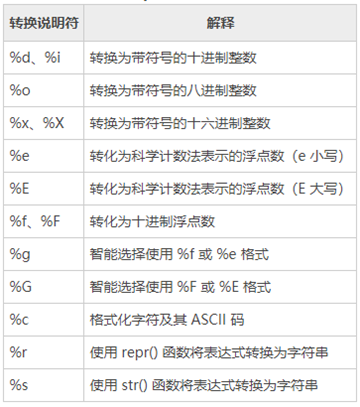There are two string formatting schemes in Python, one is the % operator that existed before Python 2.6, and the other is a new string formatting function str.format() that has been added since Python 2.6.## % Formatting Tool

Students familiar with the C printf() function will easily learn the %formatting tool. print() uses conversion specifiers beginning with % to format output for various types of data.Output of integers.

• %o – octal octal
• %d – dec decimal

Output of floating point numbers.

• %f – retains six significant digits after the decimal point. %.3f, retains 3 decimal places
• %e – retains six significant digits after the decimal point, output in exponential form. %.3e, retains three decimal places, uses scientific notation
• %g – use decimal form if six valid digits are guaranteed, otherwise use scientific notation %.3g, retaining 3 significant digits, using decimal or scientific notation

String output.

• %s – output string
• %10s – right justified, placeholder 10 bits
• %-10s – left justified, placeholder 10 bits
• %.2s – intercept 2-bit string
• %10.2s – 10-bit placeholder, two-bit string intercepted
 `````` 1 2 3 4 5 6 7 8 9 10 11 12 13 14 15 16 17 18 19 20 21 22 23 24 25 26 27 28 29 30 31 32 33 34 35 36 `````` ``````print('%o' % 20) print('%d' % 20) print('%x' % 20) print('%f' % 1.11) # 默认保留6位小数 print('%.1f' % 1.11) # 取1位小数 print('%e' % 1.11) # 默认6位小数，用科学计数法 print('%.3e' % 1.11) # 取3位小数，用科学计数法 print('%g' % 1111.1111) # 默认6位有效数字 print('%.7g' % 1111.1111) # 取7位有效数字 print('%.2g' % 1111.1111) # 取2位有效数字，自动转换为科学计数法 print('%s' % 'hello world') # 字符串输出 print('%20s' % 'hello world') # 右对齐，取20位，不够则补位 print('%-20s' % 'hello world') # 左对齐，取20位，不够则补位 print('%.2s' % 'hello world') # 取2位 print('%10.2s' % 'hello world') # 右对齐，取2位 print('%-10.2s' % 'hello world') # 左对齐，取2位 # 输出： # 24 # 20 # 14 # 1.110000 # 1.1 # 1.110000e+00 # 1.110e+00 # 1111.11 # 1111.111 # 1.1e+03 # hello world # hello world # hello world # he # he # he ``````

## format function

str.format() enhances string formatting. The basic syntax is via {} and : instead of the previous %. format function can take unlimited arguments and positions can be out of order.

Positional matching.

• without numbering, i.e. “{}”
• with numbering, i.e. “{1}”, “{2}”
• with keywords, i.e. “{a}”, “{tom}”

Format conversion.

• ‘b’ - binary. Outputs the number in base 2.
• ‘c’ - Character. Converts integers to their corresponding Unicode strings before printing.
• ’d’ - Decimal integer. Outputs the number in base 10.
• ‘o’ - Octal. Outputs the number in base 8.
• ‘x’ - Hexadecimal. Outputs numbers in base 16, with lowercase letters for digits above 9.
• ’e’ - Power notation. Print numbers in scientific notation. Use ’e’ for powers.
• ‘g’ - General format. Outputs the value in fixed-point format. When the value is particularly large, print it in power format.
• ’n’ - Numeric. Same as ’d’ when the value is an integer, same as ‘g’ when the value is a floating-point number. The difference is that it inserts a number separator depending on the region setting.
• ‘%’ - percent. Multiplies the value by 100 and prints it in fixed-point(‘f’) format, the value will be followed by a percent sign.

Left-center-right alignment and bit-completion.

• < (default) left-aligned, > right-aligned, ^ middle-aligned, = (for numbers only) to round up after the decimal point
• Take digits “{:4s}”, “{:.2f}”, etc.

Code examples and more.

 `````` 1 2 3 4 5 6 7 8 9 10 11 12 13 14 15 16 17 18 19 20 21 22 23 24 25 26 27 28 29 30 31 32 33 34 35 36 37 `````` ``````import datetime print('{} {}'.format('hello', 'world')) # 不带字段 print('{0} {1}'.format('hello', 'world')) # 带数字编号 print('{0} {1} {0}'.format('hello', 'world')) # 打乱顺序 print('{1} {1} {0}'.format('hello', 'world')) print('{a} {tom} {a}'.format(tom='hello', a='world')) # 带关键字 print('{0:b}'.format(3)) print('{:c}'.format(20)) print('{:d}'.format(20)) print('{:o}'.format(20)) print('{:x}'.format(20)) print('{:e}'.format(20)) print('{:g}'.format(20.1)) print('{:f}'.format(20)) print('{:n}'.format(20)) print('{:%}'.format(20)) print('{} and {}'.format('hello', 'world')) # 默认左对齐 print('{:10s} and {:>10s}'.format('hello', 'world')) # 取10位左对齐，取10位右对齐 print('{:^10s} and {:^10s}'.format('hello', 'world')) # 取10位中间对齐 print('{} is {:.2f}'.format(1.123, 1.123)) # 取2位小数 print('{0} is {0:>10.2f}'.format(1.123)) # 取2位小数，右对齐，取10位 print('{:<30}'.format('left aligned')) # 左对齐 print('{:>30}'.format('right aligned')) # 右对齐 print('{:^30}'.format('centered')) # 中间对齐 print('{:*^30}'.format('centered')) # 使用“*”填充 print('{:0=30}'.format(11)) # 还有“=”只能应用于数字，这种方法可用“>”代替 print('{:+f}; {:+f}'.format(3.14, -3.14)) # 总是显示符号 print('{: f}; {: f}'.format(3.14, -3.14)) # 若是+数，则在前面留空格 print('{:-f}; {:-f}'.format(3.14, -3.14)) # -数时显示-，与'{:f}; {:f}'一致 print('{:,}'.format(1234567890)) # 数字千位分隔符 print('{:%Y-%m-%d %H:%M:%S}'.format(datetime.date.today())) # 日期时间格式化 ``````

## String interpolation F-strings

In Python version 3.6.2, PEP 498 introduced a new string formatting mechanism, called “string interpolation” or more commonly known as F-strings, which provides an explicit and convenient way to embed python expressions into strings to format them.

 ``````1 2 3 4 5 6 7 8 `````` ``````# Python程序演示 # 字符串插值 n1 = 'Hello' n2 ='GeeksforGeeks' # f告诉Python恢复大括号{}中两个字符串变量名和程序的值 print(f"{n1}! This is {n2}") # 内联算法 print(f"(2 * 3)-10 = {(2 * 3)-10}") ``````

To create an f string, prefix the string with the letter " f". The string itself is formatted as str.format(). f strings provide a concise and convenient way to format python expressions embedded in string literals.

## Extension: String Templates

Template, set a string as a template, and finally get the desired string by replacing variables.

Usage examples.

 ``````1 2 3 4 5 6 7 `````` ``````from string import Template template_string = '\$who likes \$what' s = Template(template_string) d = {'who': 'Tim', 'what': 'Kong Fu'} s_out = s.substitute(d) print(s_out) ``````